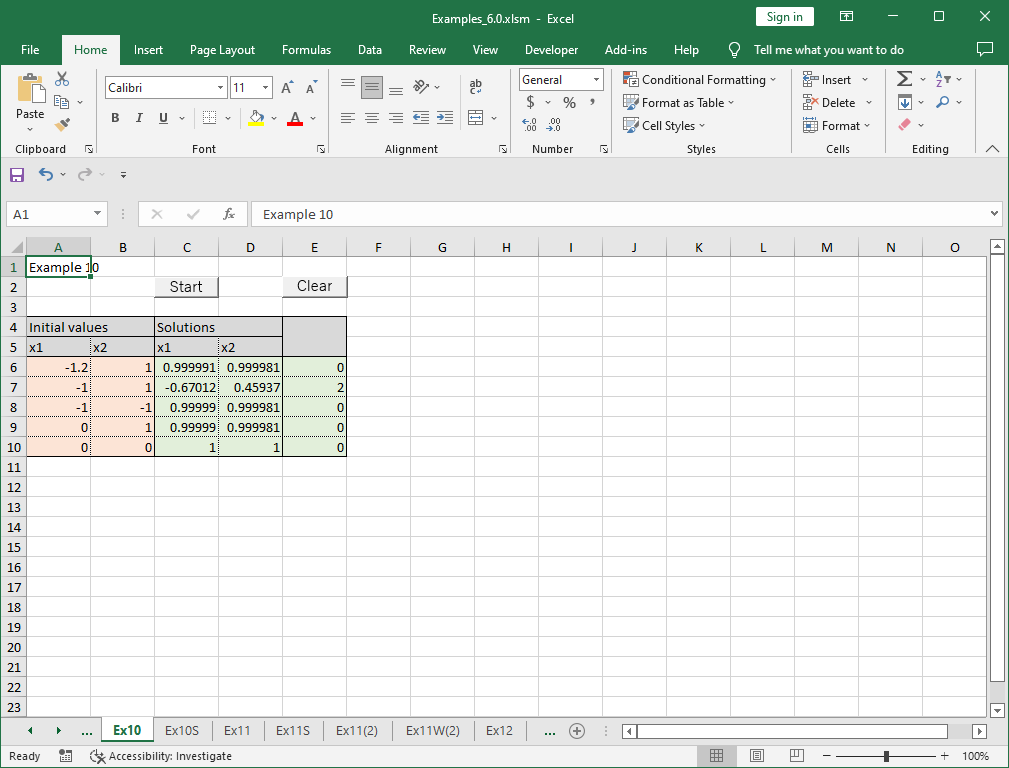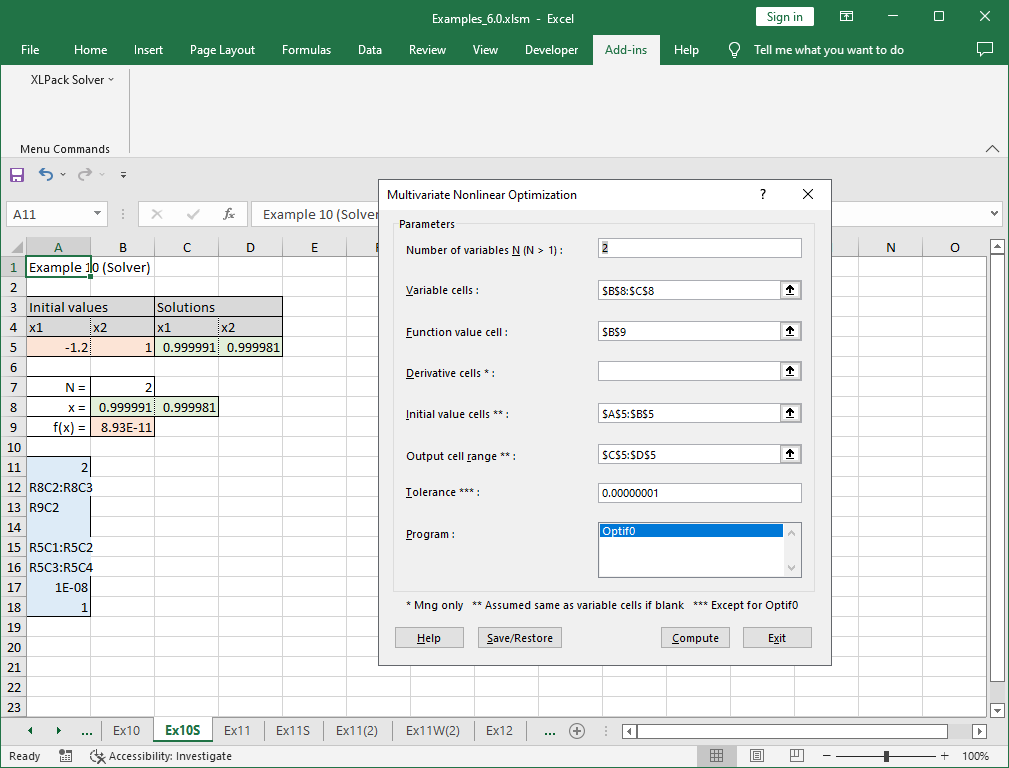# 10. Nonlinear optimization of multivariate function

Note – The example worksheets are available for download. See 1. How to obtain and use example worksheets.

Example of solving a nonlinear optimization of multivariate function, i.e. finding a minimum point of general multidimensional nonlinear function f(x1, x2, …, xn) by XLPack.

### Example

Let’s obtain the minimum point of the following function.

```
f(x1, x2) = 100(x2 - x12)2 + (1 - x1)2

```

The plot of this function is as follows (vertical axis is expressed in logarithmic value).This function is called as Rosenbrock function which is frequently used as the test problem finding minimum at (1, 1) starting from (-1.2, 1). This problem is difficult to reach the minimum point since it is in the deep bent valley.

### Solving problem with VBA program (1)

The following is an example code using VBA library subroutine Optif0.

``````Sub F(N As Long, X() As Double, Fval As Double)
Fval = 100 * (X(1) - X(0) ^ 2) ^ 2 + (1 - X(0)) ^ 2
End Sub

Sub Start()
Const NMax = 10, Ndata = 5
Dim N As Long, X(NMax) As Double, Xpls(NMax) As Double, Fpls As Double
Dim Info As Long, I As Long
N = 2
For I = 0 To Ndata - 1
'--- Input data
X(0) = Cells(6 + I, 1): X(1) = Cells(6 + I, 2)
'--- Find min point of equation
Call Optif0(N, X(), AddressOf F, Xpls(), Fpls, Info)
'--- Output result
Cells(6 + I, 3) = Xpls(0): Cells(6 + I, 4) = Xpls(1): Cells(6 + I, 5) = Info
Next
End Sub``````

You need to define the object function as the external subroutine and call Optif0 with specifying the initial value. Optif0 starts the iterative calculation from the given initial approximation and find the solution located near. The above program is designed to see what solutions are obtained when five different initial values are given.

We try five initial approximations (-1.2, 1), (-1, 1), (-1, -1), (0, 1) and (0, 0).

The following result was obtained by running this program.It did not converge for the initial approximation (-1, 1). In the above example, the difficult initial values are used since it is the test problem. But the best approximation value should be used for the real problem. There may be a problem which has multiple local minimums. In that case, the routine may converge to undesired local minimum if the best initial value is not given.

### Solving problem with VBA program (2)

The following is an example code using the reverse communication version VBA library subroutine Optif0_r.

``````Sub Start()
Const NMax = 10, Ndata = 5
Dim N As Long, X(NMax) As Double, Xpls(NMax) As Double, Fpls As Double
Dim Info As Long, I As Long
Dim XX(NMax - 1) As Double, YY As Double, IRev As Long
N = 2
For I = 0 To Ndata - 1
'--- Input data
X(0) = Cells(6 + I, 1): X(1) = Cells(6 + I, 2)
'--- Find min point of equation
IRev = 0
Do
Call Optif0_r(N, X(), Xpls(), Fpls, Info, XX(), YY, IRev)
If IRev = 1 Then YY = 100 * (XX(1) - XX(0) ^ 2) ^ 2 + (1 - XX(0)) ^ 2
Loop While IRev <> 0
'--- Output result
Cells(6 + I, 3) = Xpls(0): Cells(6 + I, 4) = Xpls(1): Cells(6 + I, 5) = Info
Next
End Sub``````

Instead of defining the object function as the external function, when returned with IRev = 1, you need to compute the object function value at XX(), enter it in YY and call Optif0_r again. For further details of reverse communication version, see here.

The same result with above is obtained by this program.

### Solving problem with Solver

“Multivariate Nonlinear Optimization” of XLPack solver add-in can be used to solve this problem. The formula (=100*(C8-B8^2)^2+(1-B8)^2) is entered in cells B9.Please refer to here for how to use solver.